Question

# le three alternatives. c. Use your findings in parts a and b to calculate the coefficient of variatio each of the three...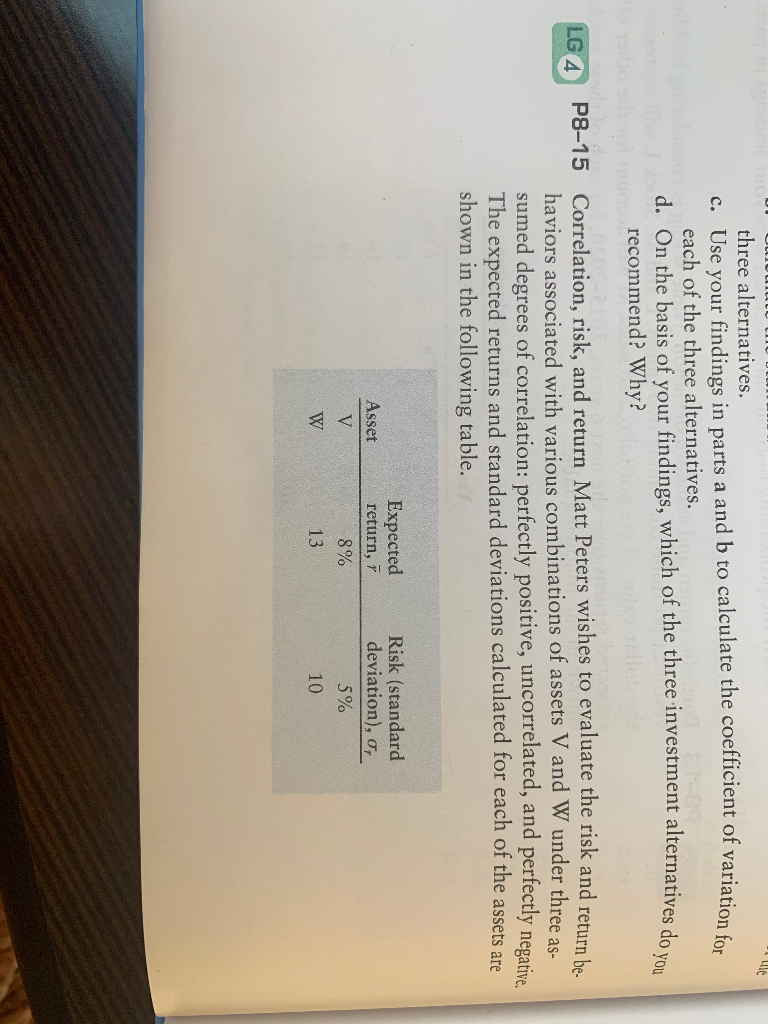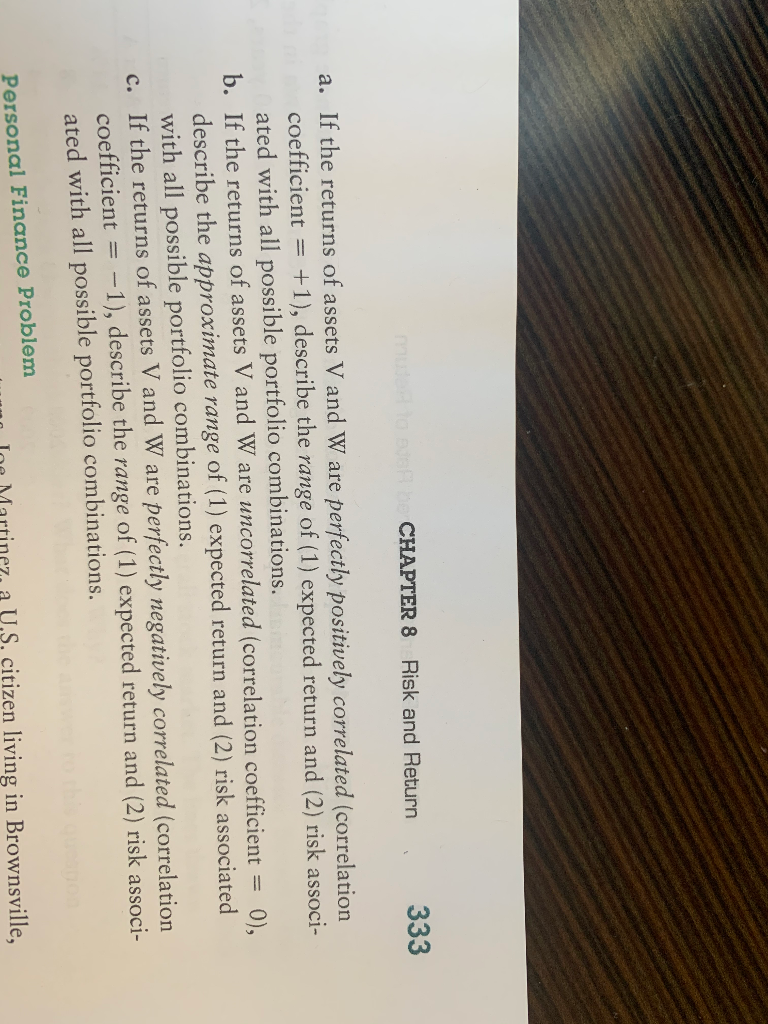le three alternatives. c. Use your findings in parts a and b to calculate the coefficient of variatio each of the three alternatives. d. On the basis of your findings, which of the three investment alternatives do recommend? Why? LG 4 P8-15 Correlation, risk, and return Matt Peters wishes to evaluate the risk and return be haviors associated with various combinations of assets V and W under three as- sumed degrees of correlation: perfectly positive, uncorrelated, and perfectly negative The expected returns and standard deviations calculated for each of the assets are shown in the following table. Asset Expected return, 7 8% 13 Risk (standard deviation), o, 5% 10 W
CHAPTER 8 Risk and Return 333 a. If the returns of assets V and W are perfectly positively correlated (correlation coefficient = +1), describe the range of (1) expected return and (2) risk associ- ated with all possible portfolio combinations. b. If the returns of assets V and W are uncorrelated (correlation coefficient = 0), describe the approximate range of (1) expected return and (2) risk associated with all possible portfolio combinations. If the returns of assets V and W are perfectly negatively correlated (correlation coefficient = -1), describe the range of (1) expected return and (2) risk associ- ated with all possible portfolio combinations. Personal Finance Problem no Ine Martinez a V.S. citizen living in Brownsville,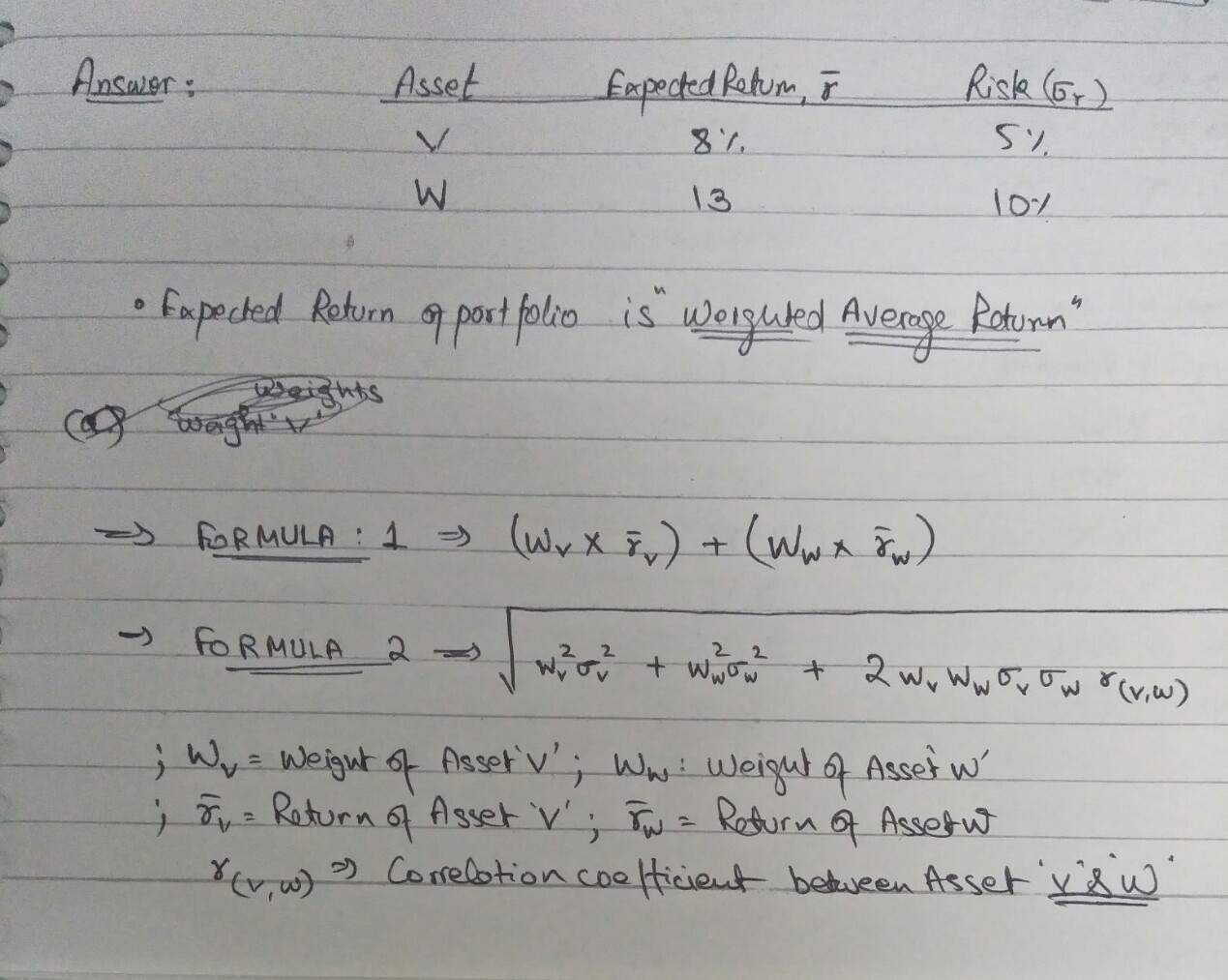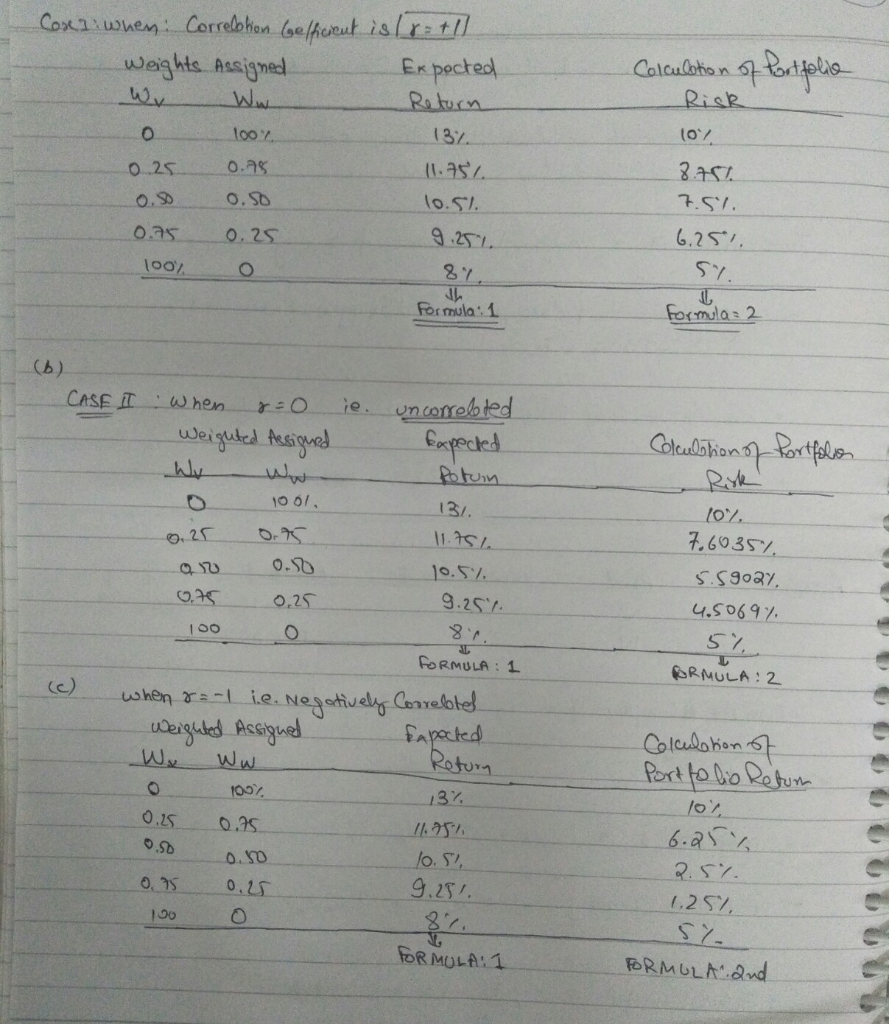#### Earn Coins

Coins can be redeemed for fabulous gifts.

Similar Homework Help Questions
• ### p8-15 A-C 333 CHAPTER 8 Risk and Return a. If the returns of assets V and...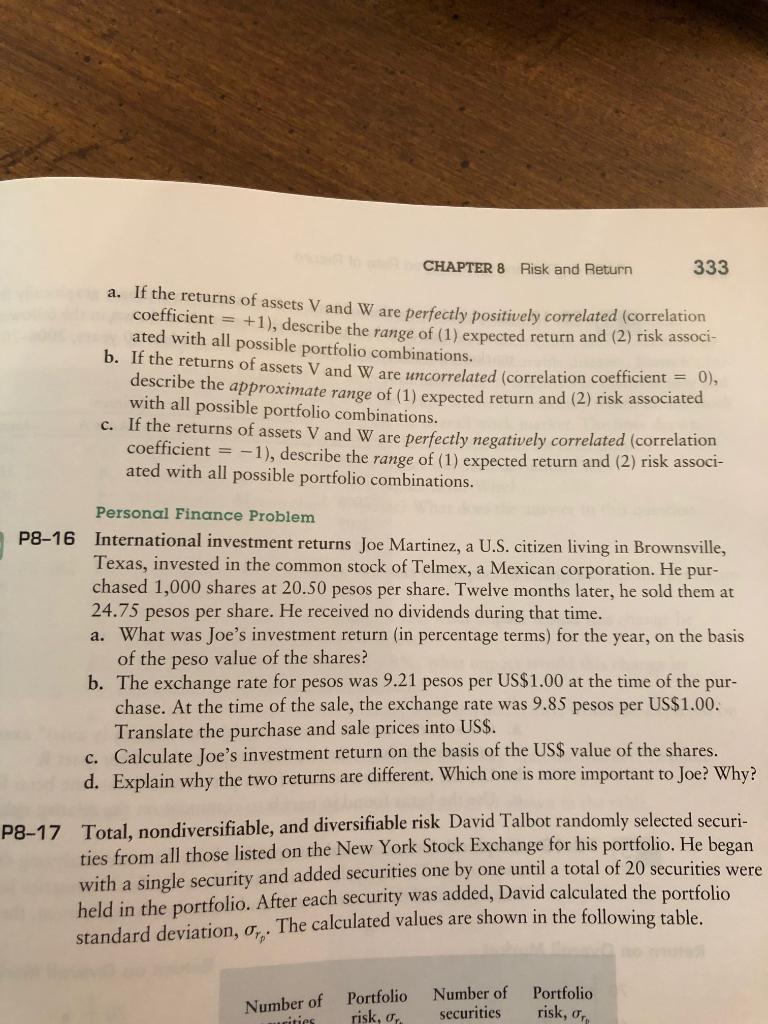p8-15 A-C 333 CHAPTER 8 Risk and Return a. If the returns of assets V and W are perfectly positively correlated (correlation coefficient = +1), describe the range of (1) expected return and (2) risk associ- ated with all possible portfolio combinations. b. If the returns of assets V and W are uncorrelated (correlation coefficient = 0), describe the approximate range of (1) expected return and (2) risk associated with all possible portfolio combinations c. If the returns of assets...

• ### LG4 5-14 Correlation, risk, and return Matt Peters wishes to evaluate the risk and return behaviors...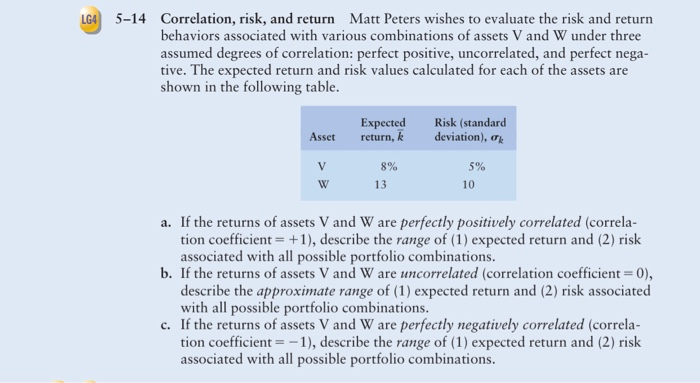LG4 5-14 Correlation, risk, and return Matt Peters wishes to evaluate the risk and return behaviors associated with various combinations of assets V and W under three assumed degrees of correlation: perfect positive, uncorrelated, and perfect nega- tive. The expected return and risk values calculated for each of the assets are shown in the following table. Asset Expected return, k Risk (standard deviation), V 5% 8% 13 a. If the returns of assets V and W are perfectly positively correlated...

• ### Assume you wish to evaluate the risk and return behaviors associated with various combinations of assets...

Assume you wish to evaluate the risk and return behaviors associated with various combinations of assets V and W under three assumed degrees of​ correlation: perfect​ positive, uncorrelated, and perfect negative. The following average return and risk values were calculated for these​ assets: Asset    Average​ Return, r Risk​ (Standard Deviation), s V 7.9​% 4.6​% W 12.7​% 9.7​% a. If the returns of assets V and W are perfectly positively correlated​ (correlation coefficient = + 1​), describe the range of​...

• ### Correlation, risk, and return Matt Peters wishes to evaluate the risk and return behaviors associated with...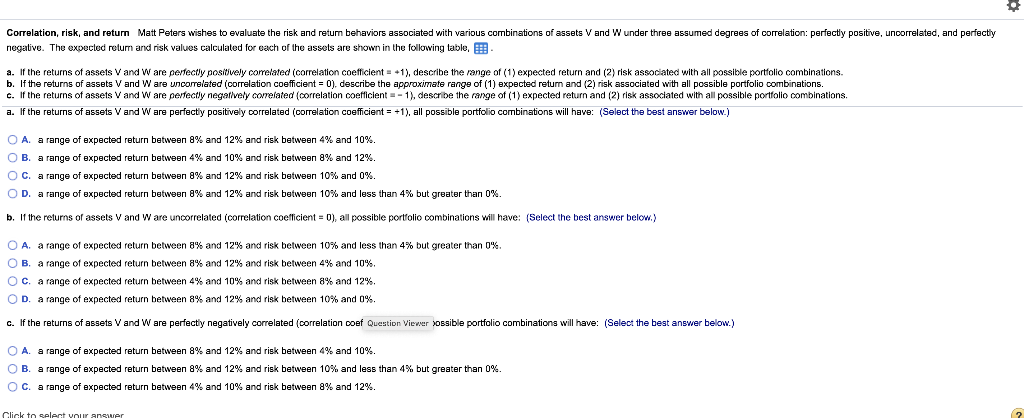Correlation, risk, and return Matt Peters wishes to evaluate the risk and return behaviors associated with various combinations of assets V and W under three assumed degrees of correlation: perfectly positive, uncorrelated, and perfectly negative. The expected return and risk values calculated for each of the assets are shown in the following table, B a. If the returns of assets V and W are perfectly positively correlated correlation coefficient = +1), describe the range of (1) expected return and (2)...

• ### Assume you wish to evaluate the risk and return behaviors associated with various combinations of two​ stocks, Alpha Sof...

Assume you wish to evaluate the risk and return behaviors associated with various combinations of two​ stocks, Alpha Software and Beta​ Electronics, under three possible degrees of​ correlation: perfect​ positive, uncorrelated, and perfect negative. The average return and standard deviation for each stock appears​ here: Asset   Average Return,overbar r                   Risk (Standard Deviation), s Alpha   5.1%   30.3% Beta                    11.2%      50.5% a. If the returns of assets Alpha and Beta are perfectly positively correlated​ (correlation coefficient equals plus 1​),...

• ### (a) Suppose that there are many stocks in the security market and that the characteristics of...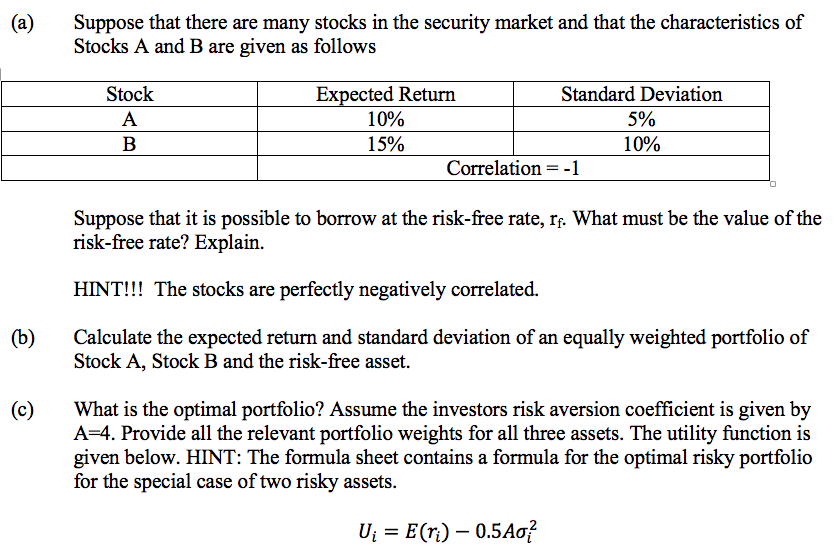(a) Suppose that there are many stocks in the security market and that the characteristics of Stocks A and B are given as follows Stock A B Expected Return Standard Deviation 10% 5% 15% 10% Correlation =-1 Suppose that it is possible to borrow at the risk-free rate, If. What must be the value of the risk-free rate? Explain. HINT!!! The stocks are perfectly negatively correlated. (b) Calculate the expected return and standard deviation of an equally weighted portfolio of...

• ### Consider the following information for three stocks, A, B, and C. The stocks' returns are positively...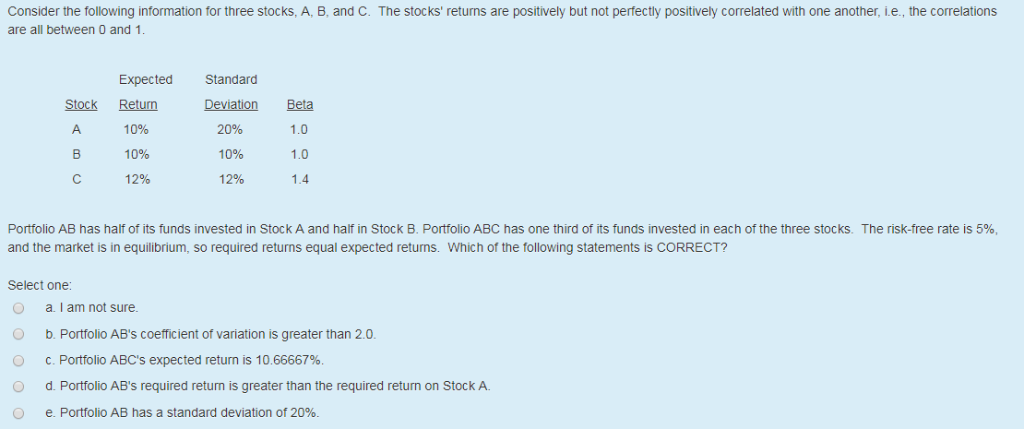Consider the following information for three stocks, A, B, and C. The stocks' returns are positively but not perfectly positively correlated with one another, i.e., the correlations are all between O and 1 ExpectedStandard Stock Retum Deviation Beta 1.0 10% 1.0 1.4 10% B10% 12% 20% 12% Portfolio AB has half of its funds invested in Stock A and half in Stock B. Portfolio ABC has one third of its funds invested in each of the three stocks. The risk-free...

• ### CAPM, PORTFOLIO RISK, AND RETURN Consider the following information for stocks A, B, and C. The...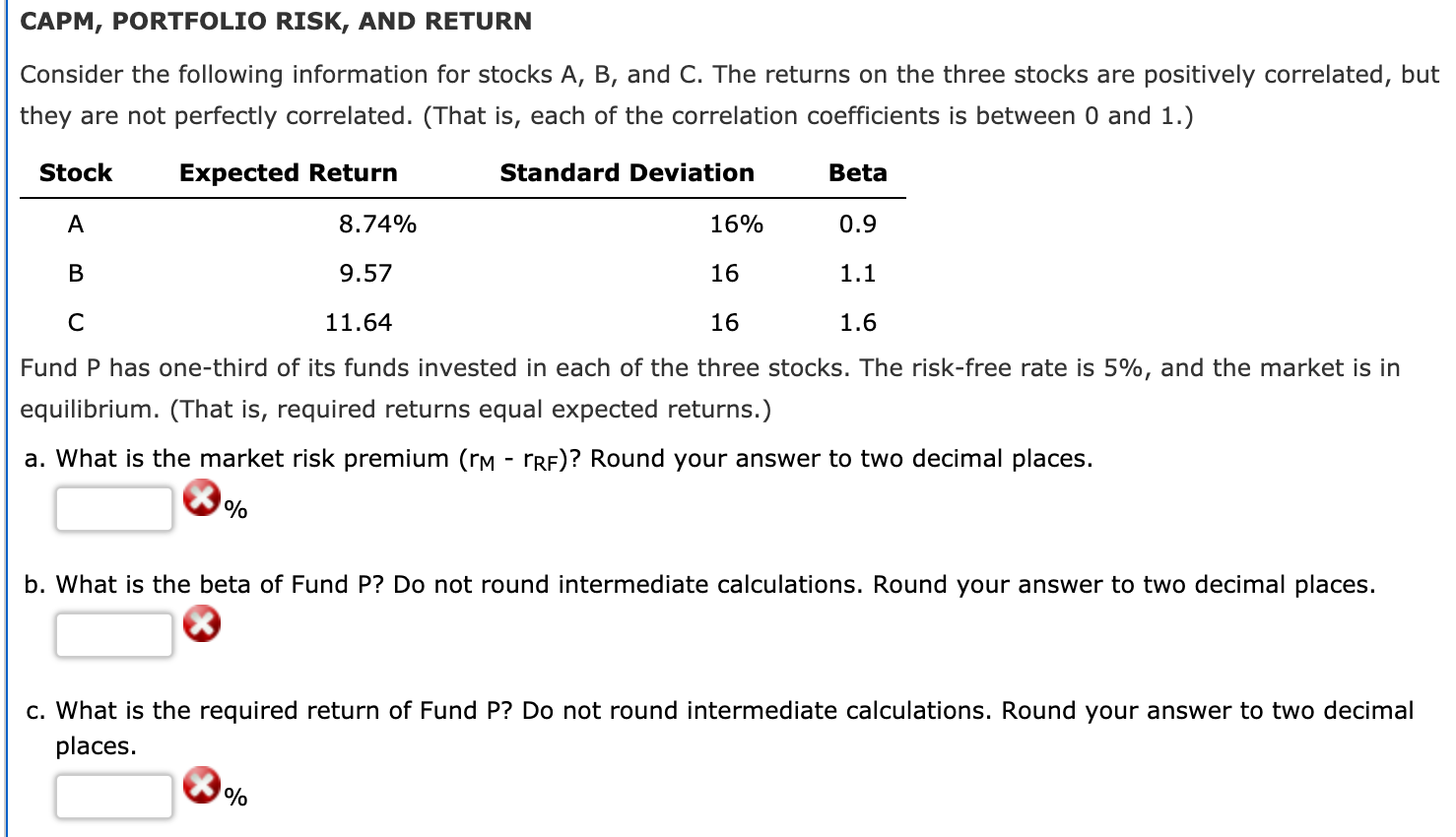CAPM, PORTFOLIO RISK, AND RETURN Consider the following information for stocks A, B, and C. The returns on the three stocks are positively correlated, but they are not perfectly correlated. (That is, each of the correlation coefficients is between 0 and 1.) Stock Expected Return Standard Deviation Beta A 8.74% 16% 0.9 B 9.57 16 1.1 C 11.64 16 1.6 Fund P has one-third of its funds invested in each of the three stocks. The risk-free rate is 5%, and...

• ### Problem 8-13 CAPM, portfolio risk, and return Consider the following information for three stocks, Stocks A, B, and C. T...

Problem 8-13 CAPM, portfolio risk, and return Consider the following information for three stocks, Stocks A, B, and C. The returns on the three stocks are positively correlated, but they are not perfectly correlated. (That is, each of the correlation coefficients is between 0 and 1.) Stock Expected Return Standard Deviation Beta A 8.30 % 16 % 0.7 B 9.90 16 1.1 C 12.30 16 1.7 Fund P has one-third of its funds invested in each of the three stocks....

• ### CAPM, portfolio risk, and return Consider the following information for three stocks, Stocks A, B...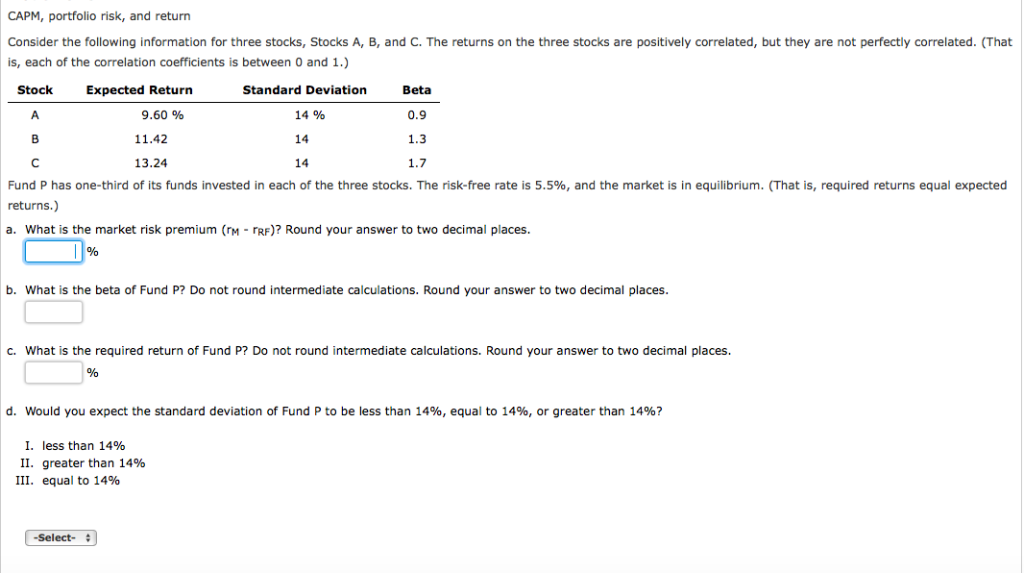CAPM, portfolio risk, and return Consider the following information for three stocks, Stocks A, B, and C. The returns on the three stocks are positively correlated, but they are not perfectly correlated. (That is, each of the correlation coefficients is between 0 and 1.) Stock Standard Deviation 14% 14 14 Beta 0.9 1.3 1.7 Expected Return 9.60 % 11.42 13.24 Fund P has one-third of its funds invested in each of the three stocks. The risk-free rate is 5.5%, and...